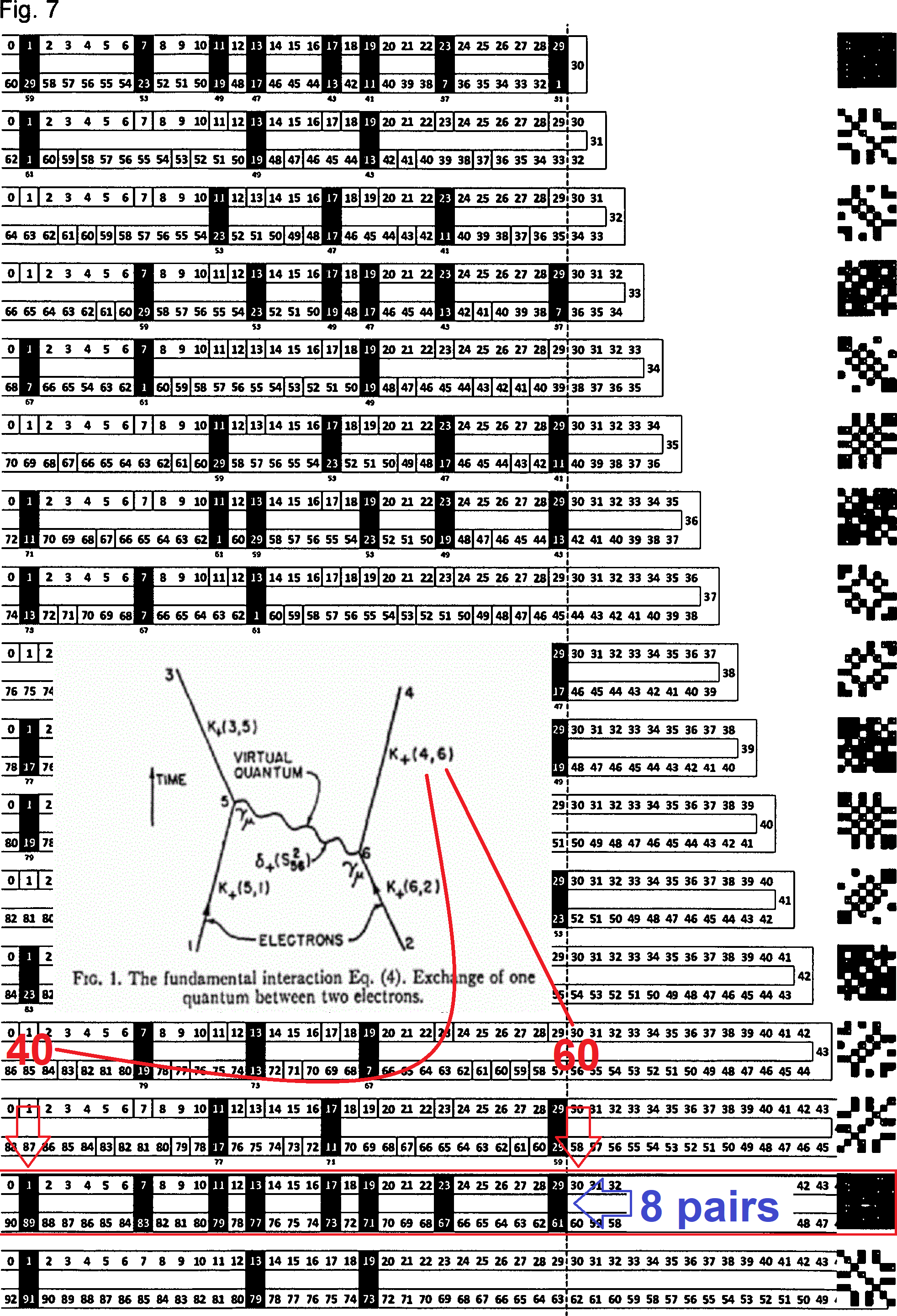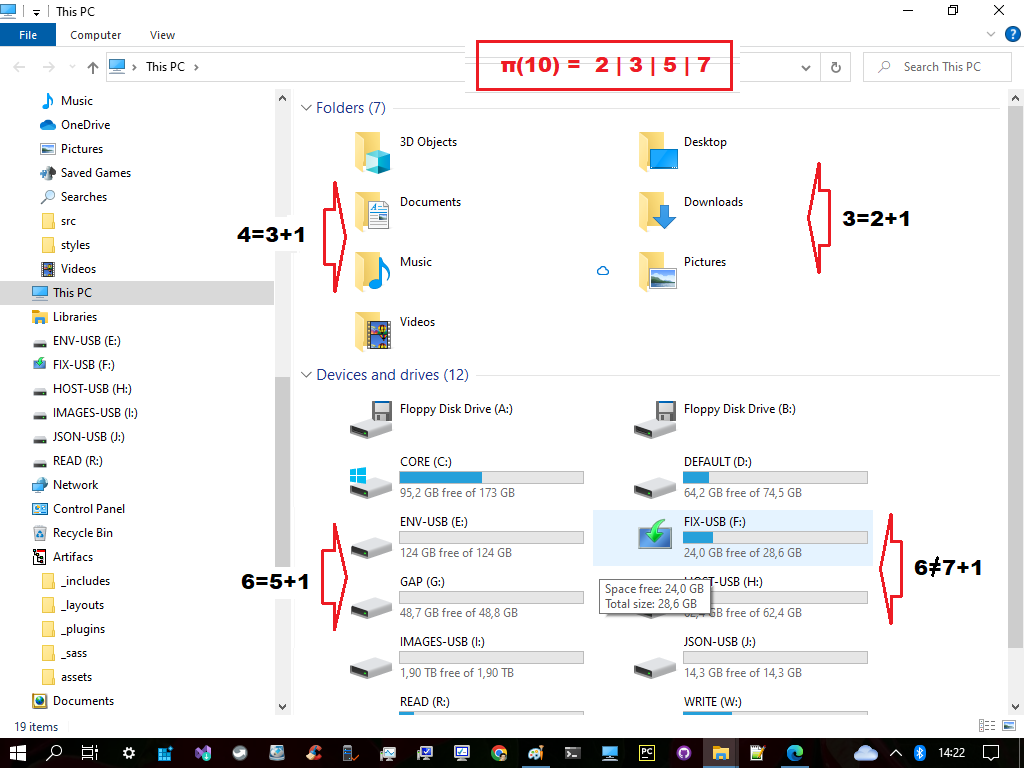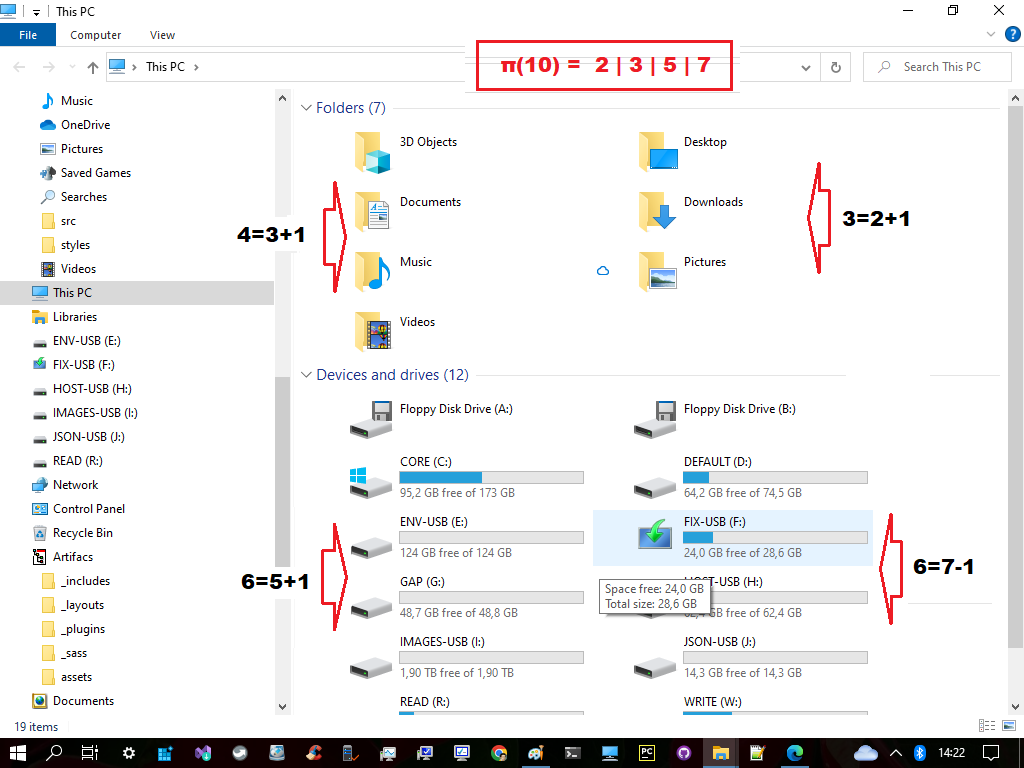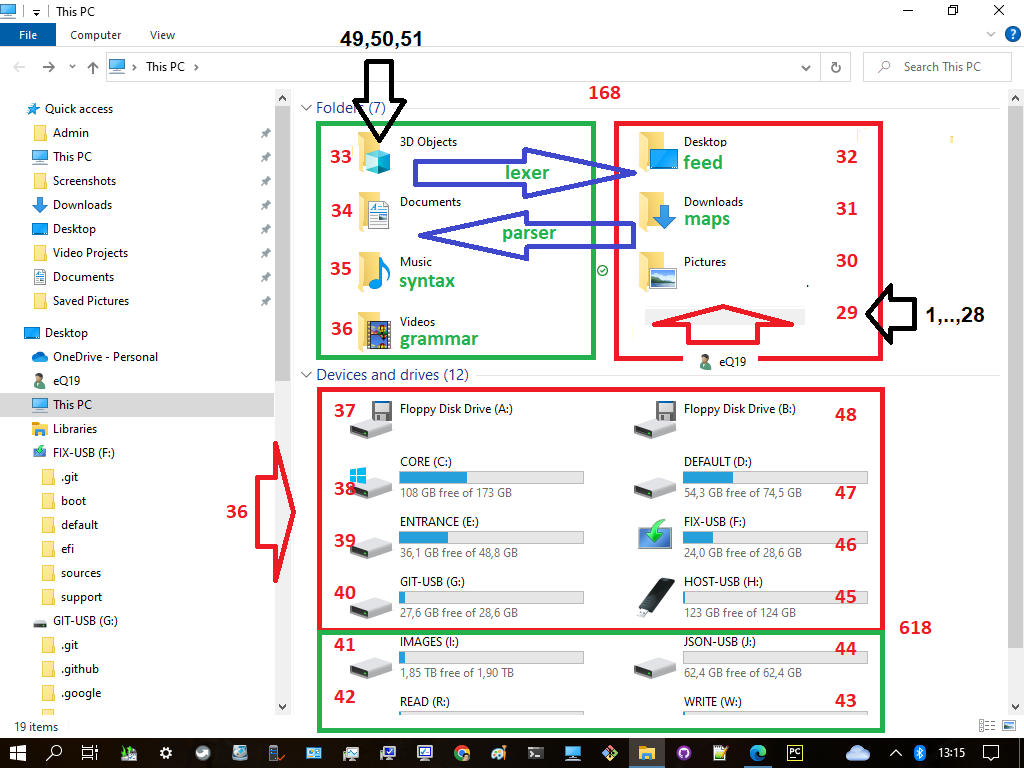# Rational vs Irrational

``````---+-----+-----
1 |  1  | 28
---+-----+-----
2 | 29  | 46
---+-----+-----
3 | 47  |{56}
---+-----+-----
4 |{57} | 61
---+-----+-----
5 | 62  | 82
---+-----+-----
6 | 83  | 94
---+-----+-----
7 | 95  | 99
---+-----+-----
8 |{100}|{123}
---+-----+-----
``````
``````           15
↓
35
↓
---+-----+-----
11 | 51  | 53   ------
---+-----+-----       |
12 | 54  | 59         |
---+-----+-----       | 6th (id:14 - id:15)
13 | 60  | 82         |
---+-----+-----       |
14 | 83  |{102} --289-¤-exponentiation zone
---+-----+-----       | 7th (id:16 - id:19)
15 |{103}| 110  ------
---+-----+-----
↓
15
↓
35
``````

Gematria is the practice of assigning a numerical value to a name, word or phrase according to an alphanumerical cipher. Single word can yield several values depending on which cipher is used. This cipher is sometimes erroneously labelled as "Jewish" or "Hebrew" by popular numerology calculators, such as Gematrix and Gematrinator.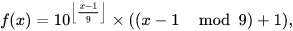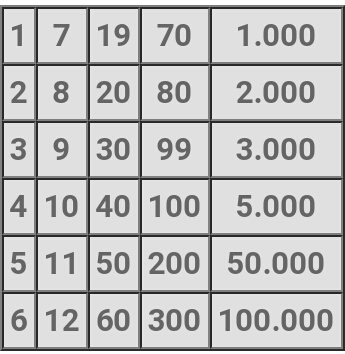It is possible that this well-known cipher was used to conceal other more hidden ciphers in Jewish texts. For instance, a scribe may discuss a sum using the ‘standard gematria' cipher, but may intend the sum to be checked with a different secret cipher.

7(111) = 1117 = 7² + 7¹ + 7° = 49 + 7 + 1 = 57In the standard (Mispar hechrechi) version of gematria, each letter is given a numerical value between 1 and 400, as shown in the following table. In the Mispar gadol variation, the five final letters are given their own values, ranging from 500 to 900.

Δ prime = 114th prime - 19 = (6 x 19)th prime - 19 = 619 - 19 = 600

``````  Sub  | i  |  β  | f
=======+====+=====+=======  ===   ===   ===   ===   ===   ===
1:1:0 | 1  |   1 | 2 {71}   1     1     |     |     |     |
-------+----+-----+-------  ---   ---    |     |     |     |
1:2:1 | 2  |   2 | 3 {71}         |     |     |     |     |
-------+----+-----+----            |     |     |     |     |
*1:2:2 | 3  |   3 | 7 = 1 + 2x3    |     |     |     |     |
-------+----+-----+----            |     |     |     |     |
*1:3:3 | 4  |   4 | 10 = 9 + 1     |     |     |     |     |
-------+----+-----+----            |     |     |     |     |
1:3:4 | 5  |   5 | 11 = 9 + 2     |     |     |     |     |
-------+----+-----+----            9     1‘    |    Δ100   |
*1:3:5 | 6  |   6 | 12 = 9 + 3     |     |     |     |     |
-------+----+-----+----            |     |     |     |     |
*1:4:6 | 7  |   7 | 13 = 9 + 4     |     |     |     |     |
-------+----+-----+----            |     |     |     |     |
1:4:7 | 8  |   8 | 14 = 9 + 5     |     |     |     |     |
-------+----+-----+----            |     |     |     |     |
*1:4:8 |{9} |   9 | 15 = 9 + 6     |     |     |     |     |
-------+----+-----+----            |     |     |     |     |
*1:4:9 |{10}|  10 | 19 = 9 + 10    |     |     |     |     |
=======+====+=====+====           ===   ---    1"   ---    |
2:1:0 | 11 |  20 | 20 = 19 + log 10¹    |     |     |     |
-------+----+-----+----                  |     |     |     |
2:2:1 | 12 |  30 | 26 = 20 + 2x3        |     |     |     |
-------+----+-----+----                  |     |     |     |
*2:2:2 | 13 |  40 | 27 = 26 + 1          |     |     |     |
-------+----+-----+----                  |     |     |     |
*2:3:3 | 14 |  50 | 28 = 26 + 2          |     |     |     |
-------+----+-----+----                  |     |     |     |
2:3:4 | 15 |  60 | 29 = 26 + 3          9‘    |   Δ200  Δ600
-------+----+-----+----                  |     |     |     |
*2:3:5 | 16 |  70 | 30 = 26 + 4          |     |     |     |
-------+----+-----+----                  |     |     |     |
*2:4:6 | 17 |  80 | 31 = 26 + 5          |     |     |     |
-------+----+-----+----                  |     |     |     |
2:4:7 |{18}|  90 | 32 = 26 + 6          |     |     |     |
-------+----+-----+----                  |     |     |     |
*2:4:8 |{19}| 100 | 36 = 26 + 10         |     |     |     |
=======+====+=====+====                 ===   ---   ---    |
*2:4:9 | 20 | 200 | 38 = 36 + log 10²          |     |     |
-------+----+-----+----                        |     |     |
3:1:0 | 21 | 300 | 40 = 36 + 2 x log 10²      |     |     |
-------+----+-----+----                        |     |     |
3:2:1 | 22 | 400 | 41 = 40 + 1                |     |     |
-------+----+-----+----                        |     |     |
*3:2:2 | 23 | 500 | 42 = 40 + 2                |     |     |
-------+----+-----+----                        |     |     |
*3:3:3 | 24 | 600 | 43 = 40 + 3                9"  Δ300    |
-------+----+-----+----                        |     |     |
3:3:4 | 25 | 700 | 44 = 40 + 4                |     |     |
-------+----+-----+----                        |     |     |
*3:3:5 | 26 | 800 | 45 = 40 + 5                |     |     |
-------+----+-----+----                        |     |     |
*3:4:6 | 27 | 900 | 46 = 40 + 6                |     |     |
-------+----+-----+----                        |     |     |
3:4:7 |{28}|1000 | 50 = 40 + 10               |     |     |
=======+====+=====+====                       ===  ====  ----
*3:4:8 |{29}|2000 | 68 = 50 + 3 x (2x3)      {10³} Δ600  Δ300
=======+====+=====+====                        Δ         ====
3:4:9 |{30}|3000 |{71}= 68 + log 10³ ---------¤         Δ900
``````

## Instaneous connection

``````  -----------------------+----+----+----+----+----+----+----+----+----+-----
True Prime Pairs Δ    |  1 |  2 |  3 |  4 |  5 |  6 |  7 |  8 |  9 | Sum
=======================+====+====+====+====+====+====+====+====+====+=====
19 → π(10)            |  2 |  3 |  5 |  7 |  - |  - |  - |  - |  - | 4th  4 x Root
-----------------------+----+----+----+----+----+----+----+----+----+-----
17 → π(20)            | 11 | 13 | 17 | 19 |  - |  - |  - |  - |  - | 8th  4 x Twin
-----------------------+----+----+----+----+----+----+----+----+----+-----
13 → π(30) → 12 (Δ1)  | 23 | 29 |  - |  - |  - |  - |  - |  - |  - |10th
=======================+====+====+====+====+====+====+====+====+====+===== 1st Twin
11 → π(42)            | 31 | 37 | 41 |  - |  - |  - |  - |  - |  - |13th
-----------------------+----+----+----+----+----+----+----+----+----+----- 2nd Twin
7 → π(60) → 19 (Δ12) | 43 | 47 | 53 | 59 |  - |  - |  - |  - |  - |17th
-----------------------+----+----+----+----+----+----+----+----+----+----- 3rd Twin
5 → π(72) → 18 (Δ13) | 61 | 67 | 71 |  - |  - |  - |  - |  - |  - |20th
=======================+====+====+====+====+====+====+====+====+====+===== 4th Twin
3,2 → 18+13+12 → 43  | 73 | 79 | 83 | 89 | 97 | 101| 103| 107| 109|29th
=======================+====+====+====+====+====+====+====+====+====+=====
Δ                                                            Δ
12+13+(18+18)+13+12   ← 36th-Δ1=151-1=150=100+2x(13+12)   ←   30th = 113 = 114-1
``````

It was as if some ghostly bridge across the city of Geneva, Switzerland, had permitted two photons of light nearly seven miles apart to respond simultaneously to a stimulus applied to just one of them.

Albert Einstein sneered at the very possibility of such a thing, calling it ‘‘spooky action at a distance.'' Scientists still (somewhat shamefacedly) speak of the ‘‘magic'' of ‘‘quantum weirdness.'' And yet all experiments in recent years have shown that Einstein was wrong and that action at a distance is real (New York Times).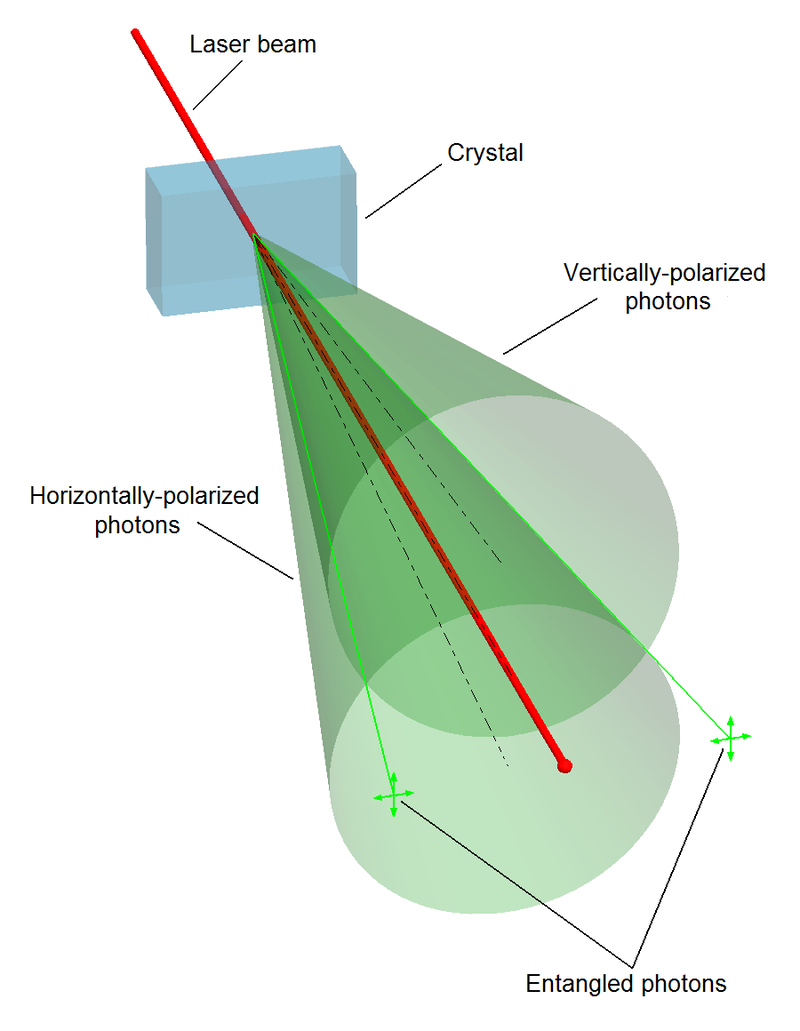``````layer | node | sub |    i     |   f
------+------+-----+----------+-----+-----+-----+                                    ---
|      |     |    1,2:1 |   1 |  30 |  40 | 71 (2,3) ‹-------------------       |
|      |  1  +----------+-----+-----+-----+                              |      |
|  1   |     |        2 |                                                |      5¨  encapsulation
|      |-----+----------+            -----------------------------       |      |
|      |     |        3 |           |                             |      |      |
1   +------+  2  +----------+----       |       LAGGING SCHEME        |      |     ---
|      |     |        4 |           |    (Exponentiation Zone)    |      |      |
|      +-----+----------+           |                             |      |      |
|  2   |     |        5 |           ------------------------------       |      7¨  abstraction
289   |      |  3  +----------+                                                |      |
|     |      |     |        6 |  ‹---------------------------- Φ               | {6®} |
------+------+-----+----------+-----+-----                                     |     ---
|      |     |     11:7 |   5 |   9 |  14 (20) --------› ¤               |      |
|      |  4  +----------+-----+-----+-----+                              |      |
|  3   |     |     12:8 |   9 |  60 |  40 | 109 (26) «------------       |     11¨  polymorphism
|      +-----+----------+-----+-----+-----+                       |      |      |
|      |     |     13:9 |   9 |  60 |  69 (27) «-- Δ19 (Rep Fork) | {2®} |      |
2   +------|  5  +----------+-----+-----+-----+                       |      |     ---
|      |     |    14:19 |   9 |  60 |  40 | 109 (28) -------------       |      |
|      |-----+----------+-----+-----+-----+                              |      |
|  4   |     | 15,18:11 |   1 |  30 |  40 | 71 (29,30,31,32) ------------      13¨  inheritance
329   |      |  6  +----------+-----+-----+-----+                                     |
|     |      |     |    19:12 |  10 |  60 | {70} (36) -------› Φ                      |
------+------+-----+----------+-----+-----+                                          ---
``````

The twin-photon experiment by Dr. Nicolas Gisin of the University of Geneva and his colleagues was the most spectacular demonstration yet of the mysterious long-range connections that exist between quantum events, connections created from nothing at all, which in theory can reach instantaneously from one end of the universe to the other.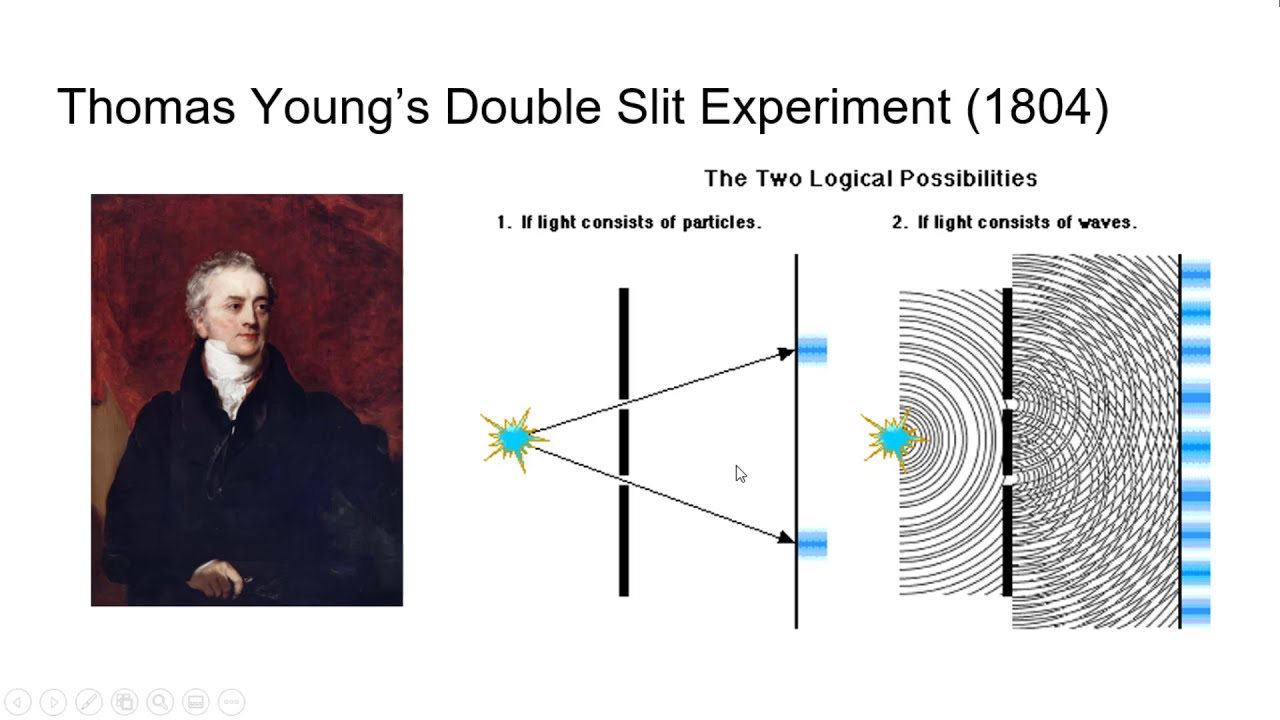According to quantum mechanics, particles are simultaneously in two or more states until observed – an effect vividly captured by Schrödinger's famous thought experiment of a cat that is both live and dead simultaneosly.

Importantly, there is also no conflict with special relativity, which forbids faster-than-light communication. The fact that measurements over vast distances are correlated does not imply that information is transmitted between the particles. Two parties far apart performing measurements on entangled particles cannot use the phenomenon to pass along information faster than the speed of light. (Astronomy).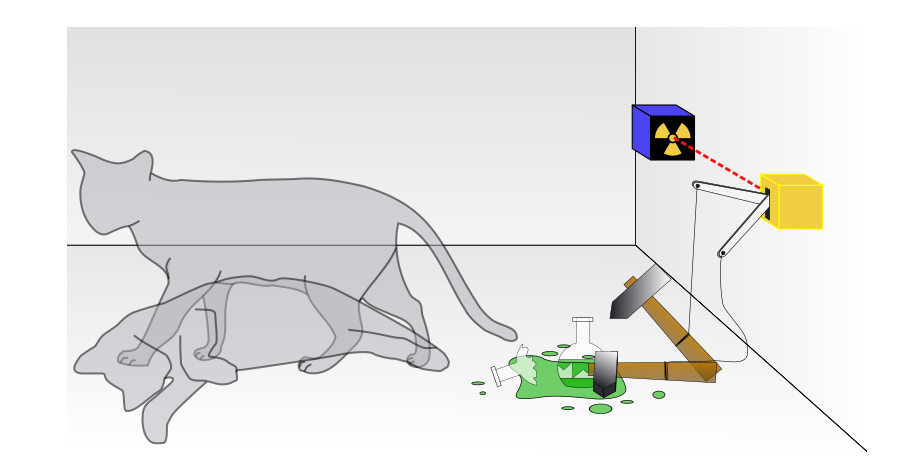### Dark Energi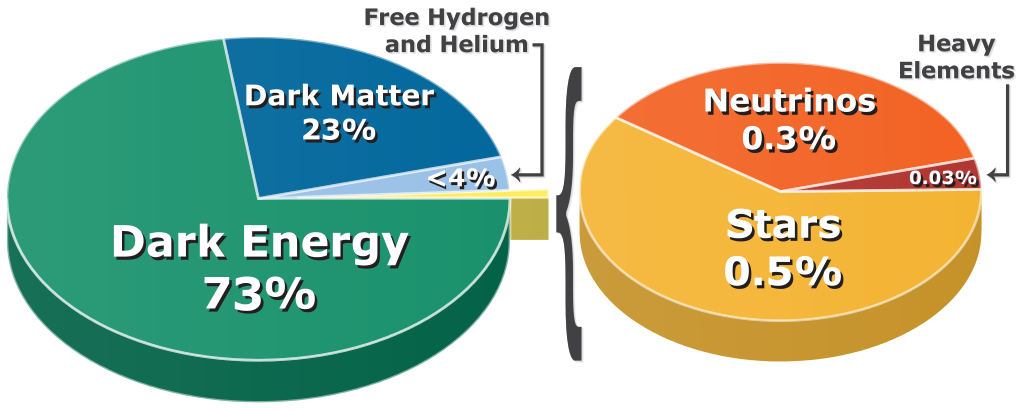Our results show that about 69% of our universe's energy is dark energy. They also demonstrate, once again, that Einstein's simplest form of dark energy – the cosmological constant – agrees the most with our observations (The Conversation).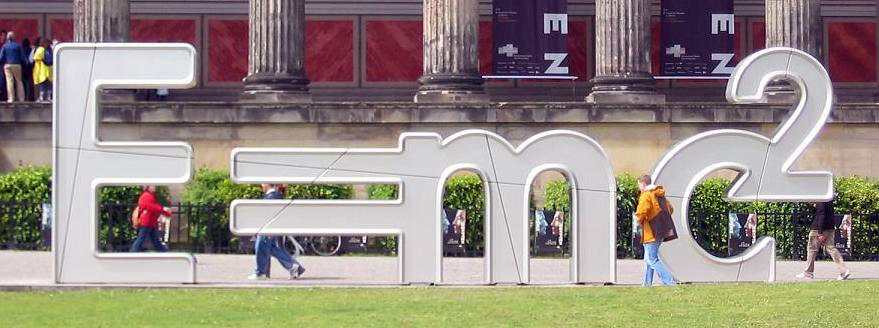Many physicists aren't satisfied with this explanation, though. They want a more fundamental description of its nature. Is it some new type of energy field or exotic fluid? Or is it a sign that Einstein's equations of gravity are somehow incomplete? What's more, we don't really understand the universe's current rate of expansion (The Conversation).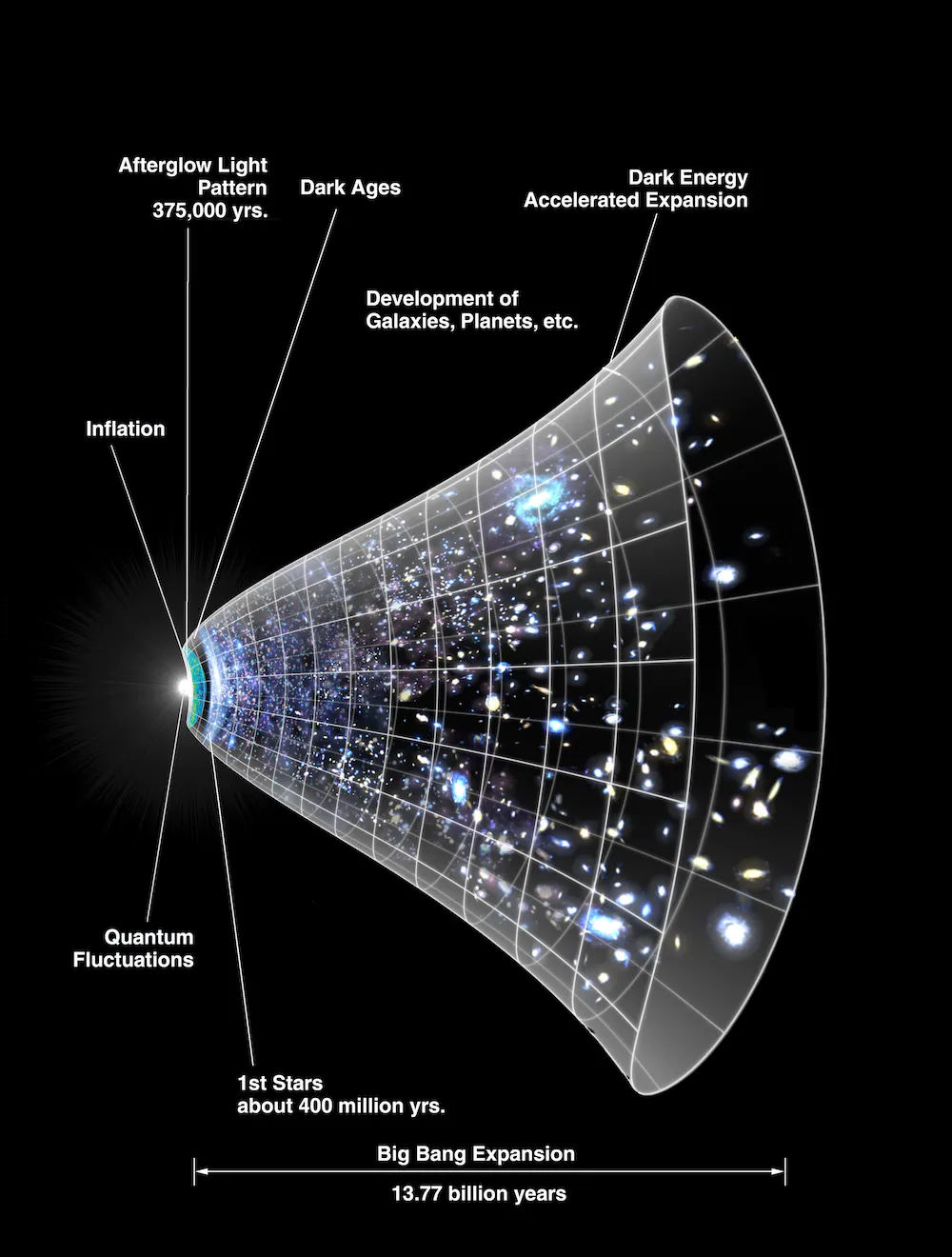The multiverse is a hypothetical group of multiple universes. Together, these universes comprise everything that exists: the entirety of space, time, matter, energy, information, and the physical laws and constants that describe them. The different universes within the multiverse are called "parallel universes", "other universes", "alternate universes" (Wikipedia).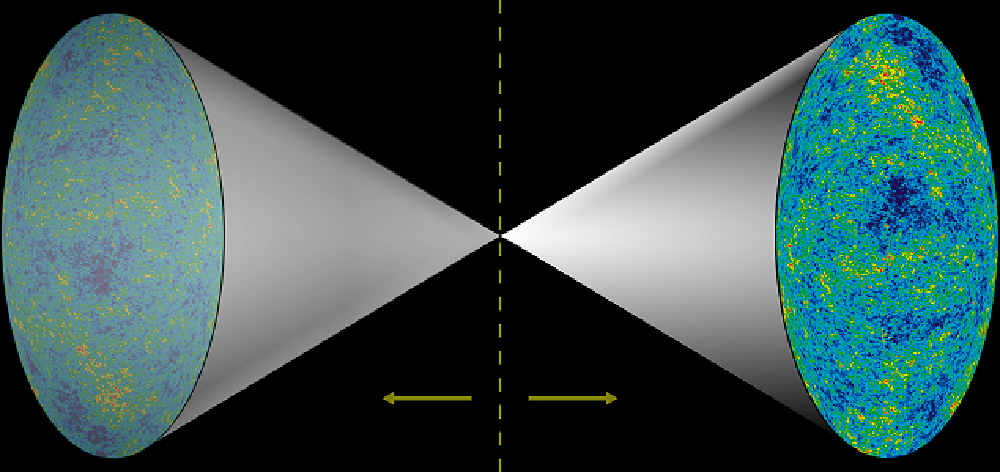102 = 2 + 100 = 2 + 60 + 40